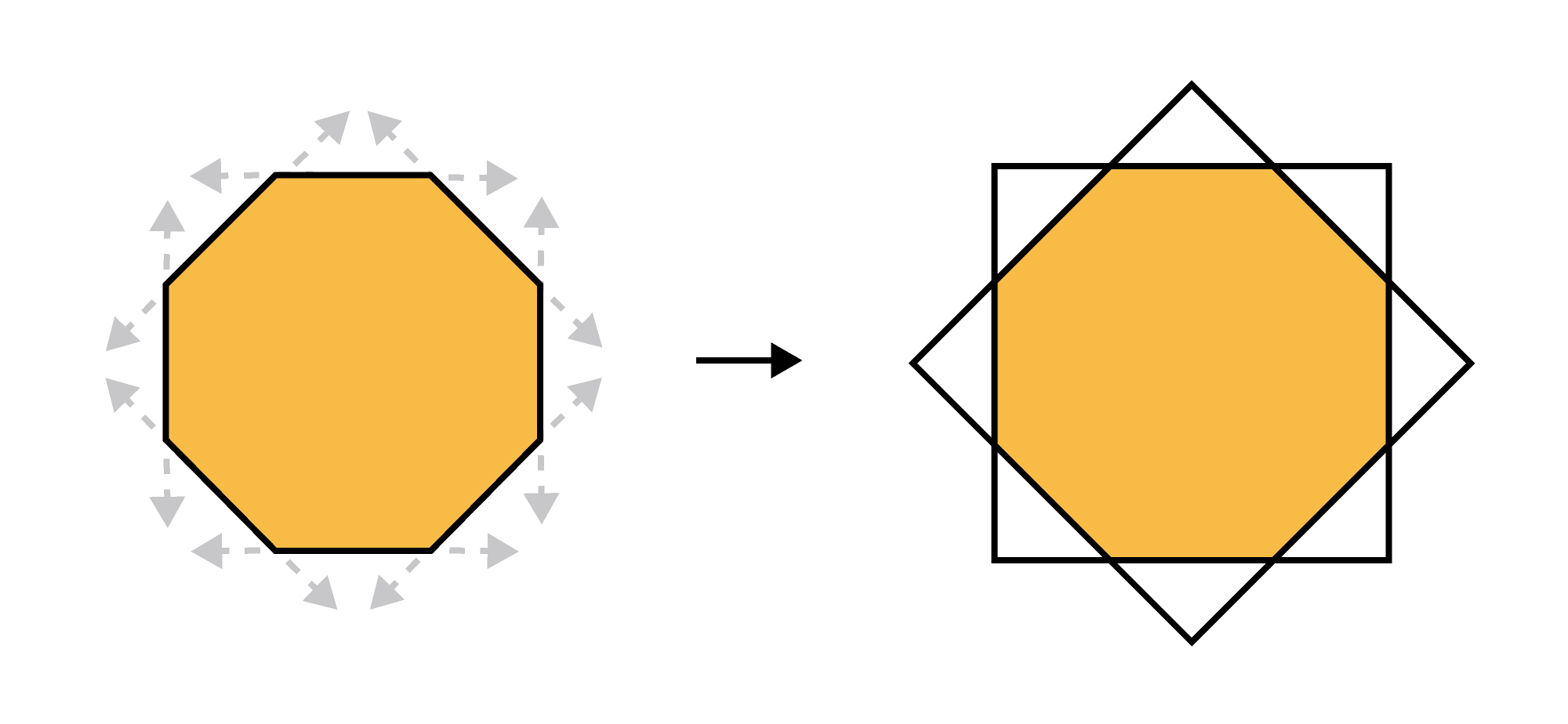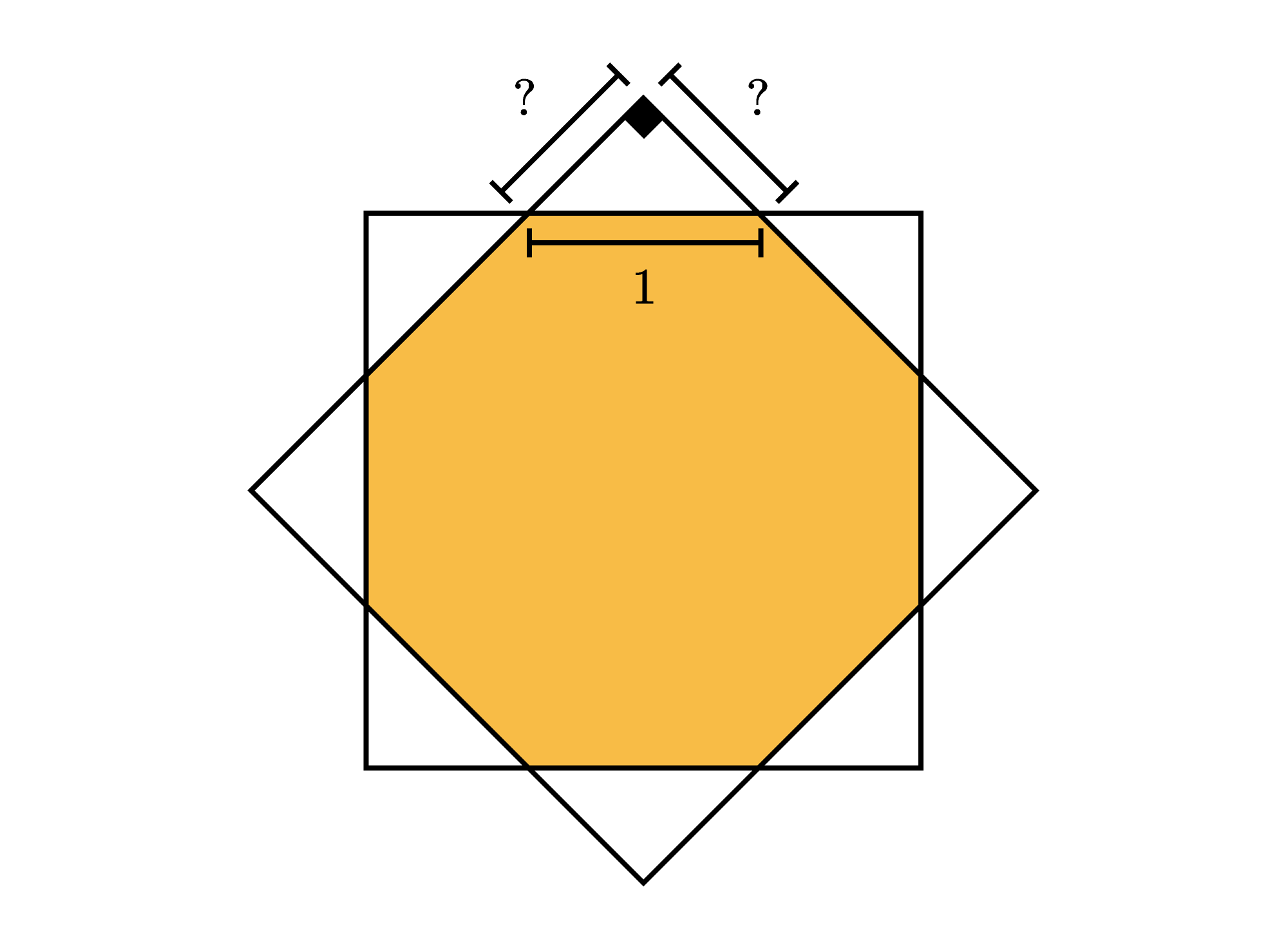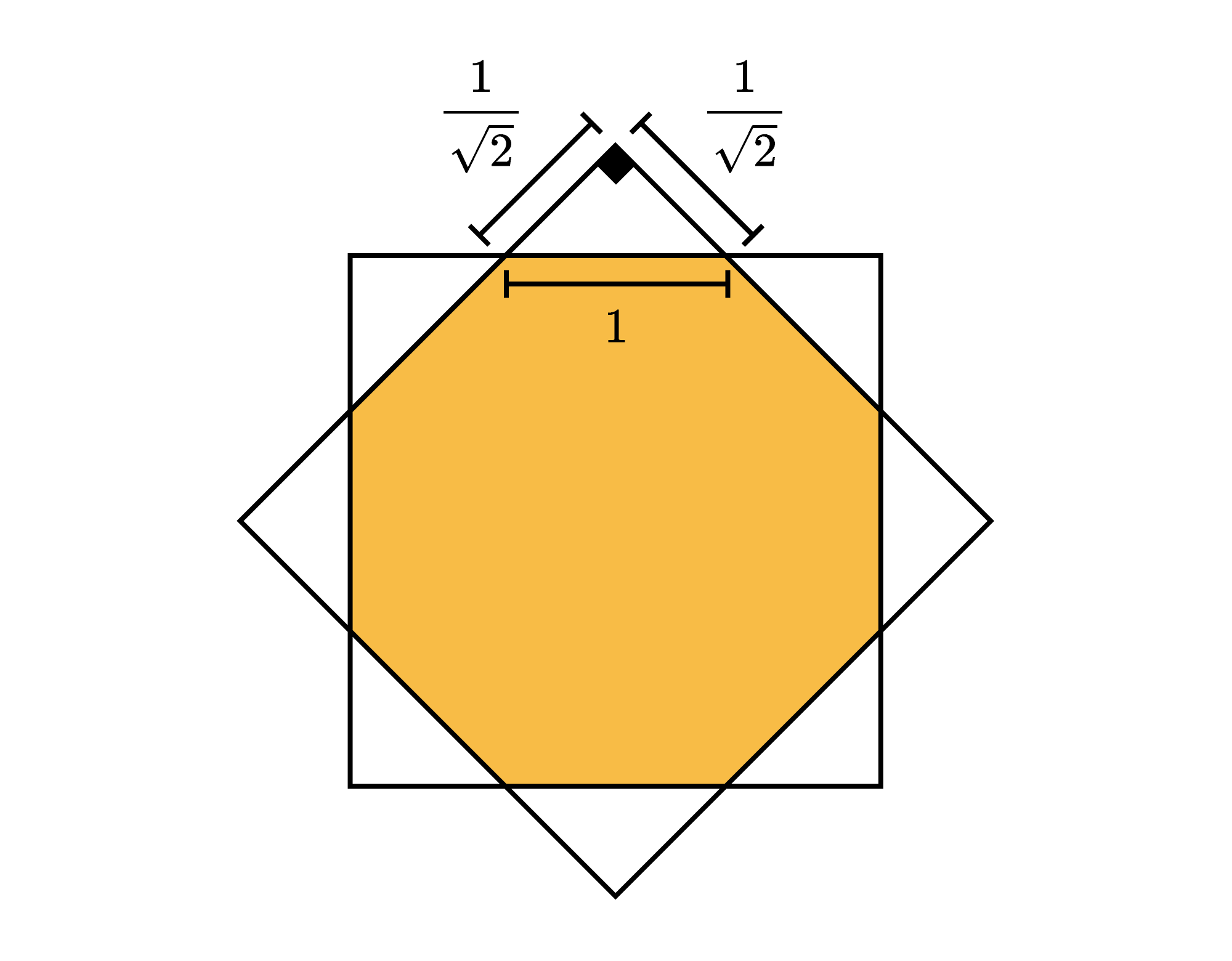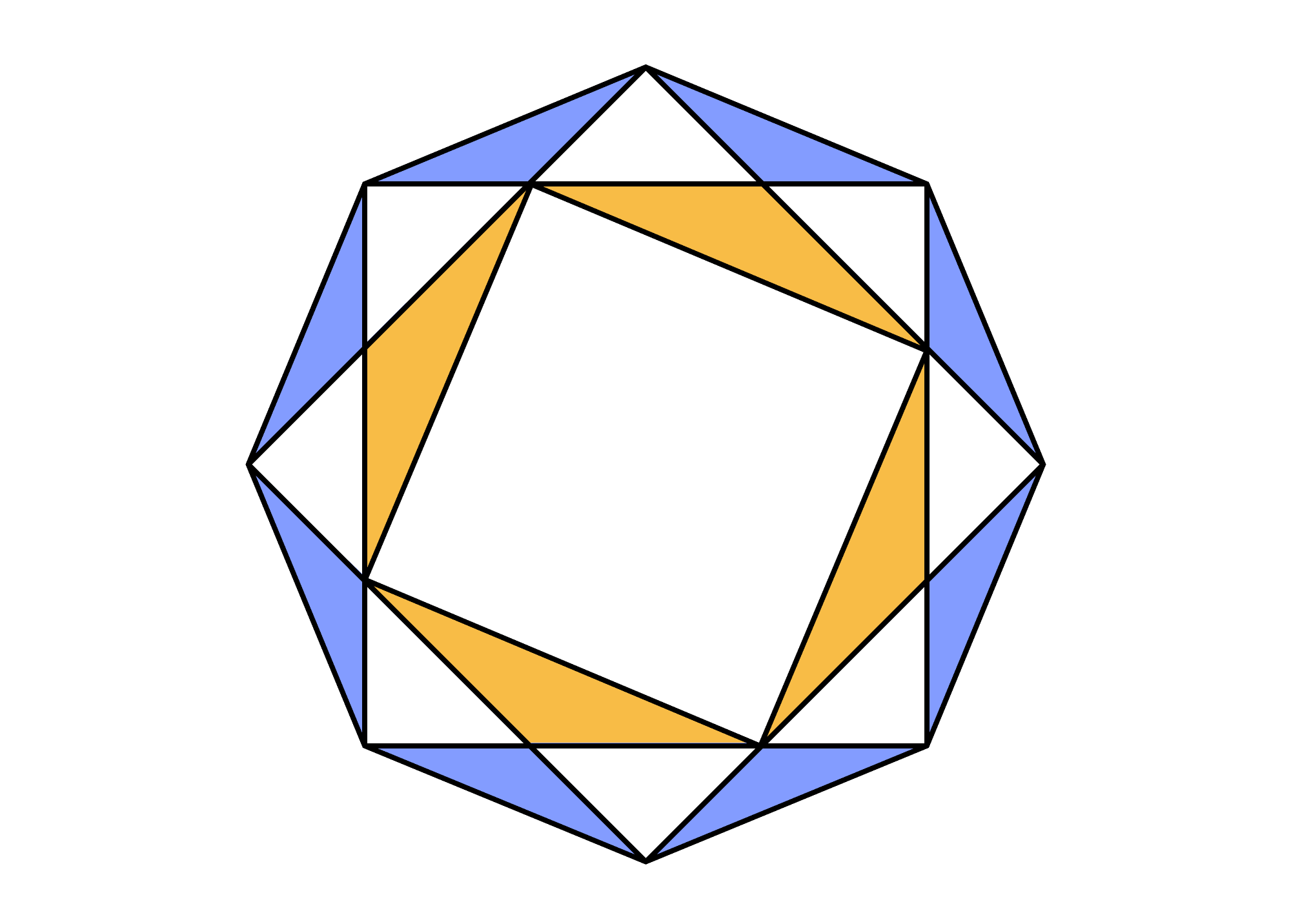## Octagons and Squares

By extending the sides of a regular polygon like this octagon, we can make a star.We call this star a stellation of the polygon. How do the side lengths of the octagon and the stellation compare? How do their perimeters compare? Keep reading to find out, or dive right into the challenge for a more extended question.

Let's see if we can use the symmetry of both figures to help. All of the side lengths of the octagon are equal, and so are the side lengths of the stellation. That cuts down on our work since, by finding one side length of either shape, we've found every side length of the shapes.

Let's label the length of one of the octagon's sides as $1.$ To which side (or sides) of the stellation should we relate this side?The octagon's side forms a right triangle with two sides of the stellation. Those other two sides have equal lengths, so our right triangle is isosceles. That makes it a $45^\circ\text{-}45^\circ\text{-}90^\circ$ right triangle.

The hypotenuse of such a triangle is $\sqrt2$ times as long as either leg. This means the two legs of our triangle each have a length of $1 \div \sqrt2 = \frac{1}{\sqrt2}.$The octagon's sides are $\mathit{\sqrt2}$ times as long as the stellation's sides.

The stellation has twice as many sides, though, so its perimeter is \begin{aligned} 2 \times \frac{1}{\sqrt2} & = \frac{2}{\sqrt2} = \sqrt2 \end{aligned} times as long as the octagon's perimeter.

Does either of these facts help with the challenge below?

# Today's Challenge

The figure below is made of regular octagons and squares.Which triangles have the greater total area?